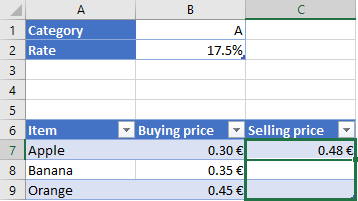﻿ Excel formula - meaning of at sign @ and dollar \$ in function

# Excel formula - meaning of at sign @ and dollar \$ in function

Hello, what is the meaning of at sign @ and dollar sign \$ in excel formula ?

For example what is the difference between function =sum(A2:A10) and =sum(\$A\$2:\$A\$10) ? It looks it gives the same results.
Hi,

The at sign is usually used as reference to the cell in the named column at the current row. For example when you want to multiply values in inserted Table 1 with headers Unit price and Units sold:

=C3*D3 will work the same as =Table1[@[Unit price]]*Table1[@[Units sold]]

The dollar sign is usually used to indicate absolute reference. For example when the formula is moved or copied to other cells and you need to use fixed position of specific cell.

Formula in C7 is =\$B\$2+1*B7. When you will copy it to C8, it will change to =\$B\$2+1*B8, so the rate from B2 is still used, because of absolute reference is set with dollar signs:When you don't use the absolute reference for B2 and you will copy the formula to C8, it will change to =B3+1*B8, so it will not provide expected correct result.
Related questions